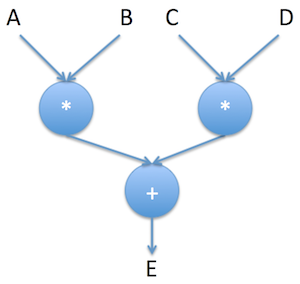@liuhui0803 2017-05-13T08:44:57.000000Z 字数 3897 阅读 1516

# MXNet API入门 —第2篇

机器学习 深度学习 神经网络 MXNet AWS

Apache MXNet是一种功能全面、可以灵活编程并且扩展能力超强的深度学习框架，支持包括卷积神经网络(CNN)与长短期记忆网络(LSTM)在内的顶尖深度模型。这一系列文章介绍了MXNet的基本概念和使用方法。本篇主要介绍了MXNet的Symbol API。

## 计算步骤？是说代码吗？## 数据流编程E = (A*B) + (C*D)

A、B、C、D具体是什么目前暂不重要，它们实际上是符号（Symbol）

## Symbol API

>>> import mxnet as mx>>> a = mx.symbol.Variable('A')>>> b = mx.symbol.Variable('B')>>> c = mx.symbol.Variable('C')>>> d = mx.symbol.Variable('D')>>> e = (a*b)+(c*d)

>>> (a,b,c,d)(<Symbol A>, <Symbol B>, <Symbol C>, <Symbol D>)>>> e<Symbol _plus1>>>> type(e)<class 'mxnet.symbol.Symbol'>

A、B、C、D是我们明确声明的符号。但E略有不同，它也是符号，但实际上它是“+”运算的结果。接下来进一步看看E。

>>> e.list_arguments()['A', 'B', 'C', 'D']>>> e.list_outputs()['_plus1_output']>>> e.get_internals().list_outputs()['A', 'B', '_mul0_output', 'C', 'D', '_mul1_output', '_plus1_output']

• E取决于变量A、B、C、D，
• 用于计算E的是一次求和操作，
• E实际上就是 (a*b)+(c*d) 的结果。

## NDArray与Symbol的绑定

>>> import numpy as np>>> a_data = mx.nd.array(, dtype=np.int32)>>> b_data = mx.nd.array(, dtype=np.int32)>>> c_data = mx.nd.array(, dtype=np.int32)>>> d_data = mx.nd.array(, dtype=np.int32)

>>> executor=e.bind(mx.cpu(), {'A':a_data, 'B':b_data, 'C':c_data, 'D':d_data})>>> executor<mxnet.executor.Executor object at 0x10da6ec90>

>>> e_data = executor.forward()>>> e_data[<NDArray 1 @cpu(0)>]>>> e_data<NDArray 1 @cpu(0)>>>> e_data.asnumpy()array(, dtype=int32)

>>> a_data = mx.nd.uniform(low=0, high=1, shape=(1000,1000))>>> b_data = mx.nd.uniform(low=0, high=1, shape=(1000,1000))>>> c_data = mx.nd.uniform(low=0, high=1, shape=(1000,1000))>>> d_data = mx.nd.uniform(low=0, high=1, shape=(1000,1000))>>> executor=e.bind(mx.cpu(), {'A':a_data, 'B':b_data, 'C':c_data, 'D':d_data})>>> e_data = executor.forward()>>> e_data[<NDArray 1000x1000 @cpu(0)>]>>> e_data<NDArray 1000x1000 @cpu(0)>>>> e_data.asnumpy()array([[ 0.89252722,  0.46442914,  0.44864511, ...,  0.08874825,         0.83029556,  1.15613985],       [ 0.10265817,  0.22077513,  0.36850023, ...,  0.36564362,         0.98767519,  0.57575727],       [ 0.24852338,  0.6468209 ,  0.25207704, ...,  1.48333383,         0.1183901 ,  0.70523977],       ...,       [ 0.85037285,  0.21420079,  1.21267629, ...,  0.35427764,         0.43418071,  1.12958288],       [ 0.14908466,  0.03095067,  0.19960476, ...,  1.13549757,         0.22000578,  0.16202438],       [ 0.47174677,  0.19318949,  0.05837669, ...,  0.06060726,         1.01848066,  0.48173574]], dtype=float32)

• 我们可以使用自己已经很熟悉的指令式编程模式加载和准备数据，甚至可以在这个过程中使用外部库（整个过程和传统变成方式完全相同）。
• 计算过程则使用符号式编程方式进行，借此MXNet不仅可以实现代码与数据的解耦，而且可以随着Graph的优化实现并行执行。

- 第3篇：Module API
- 第4篇：使用预训练模型进行图片分类（Inception v3）
- 第5篇：进一步了解预训练模型（VGG16和ResNet-152）
- 第6篇：通过树莓派进行实时物体检测（并让它讲话！）• 私有
• 公开
• 删除